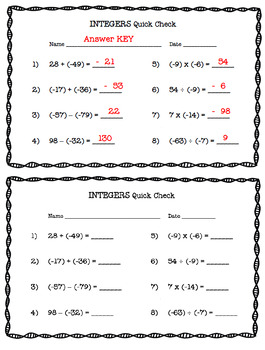Subject
Resource Type
File Type

Zip

(744 KB|2 pages)
Product Rating
Standards
• Product Description
• StandardsNEW

Attention Math Teachers ~ Do you want a quick and easy way to assess your students' ability to add, subtract, multiply, and divide integers? Well this Quick Check is what you need! These are printed 2 per page to save paper. Answer KEY included. You'll enjoy using this year after year!

Be sure to L@@K at my other, 675+ TERRIFIC teaching resources!

~ ~ THANK YOU KINDLY ~ ~

Solve real-world and mathematical problems involving the four operations with rational numbers.
Apply properties of operations as strategies to multiply and divide rational numbers.
Understand that integers can be divided, provided that the divisor is not zero, and every quotient of integers (with non-zero divisor) is a rational number. If 𝘱 and 𝘲 are integers, then –(𝘱/𝘲) = (–𝘱)/𝘲 = 𝘱/(–𝘲). Interpret quotients of rational numbers by describing real-world contexts.
Apply and extend previous understandings of multiplication and division and of fractions to multiply and divide rational numbers.
Understand subtraction of rational numbers as adding the additive inverse, 𝘱 – 𝘲 = 𝘱 + (–𝘲). Show that the distance between two rational numbers on the number line is the absolute value of their difference, and apply this principle in real-world contexts.
Total Pages
2 pages
Included
Teaching Duration
N/A
Report this Resource to TpT
Reported resources will be reviewed by our team. Report this resource to let us know if this resource violates TpT’s content guidelines.